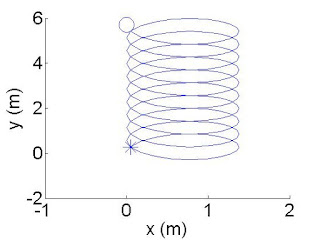## Friday, August 5, 2016

Now that you understand why a proton or electron from the Sun that bombards the Earth's magnetic field lines undergoes cyclotron motion, let's make things slightly more complicated.  Now, the magnetic fields that we'll deal with have a gradient.  Simply put, this means that the magnetic field line is stronger on one end than on the other sections of the magnetic field line.  In mathematical terms, the magnetic field vector $$\textbf{B}$$ is no longer constant with respect to position; thanks to the gradient, changes in $$\textbf{B}$$ only depend on location.  (For now, this vector is only a function of position--it remains constant over time.)   Just to clarify, I'll add that the Lorentz force--discussed in the previous post--applied by the $$\textbf{B}$$ field onto the particle is dependent on the direction, or angle with respect to the $$\textbf{B}$$ field, of the particle's velocity, but this force is not dependent on the location of the particle while it is inside of that magnetic field as long as that magnetic field has no gradient.
Pheww!! ... tough explanation ... it can be hard sometimes to explain the concept of vectors in contrast to vectors that are functions of position to people that might not utilize vector mathematics in their everyday lives.

The simulation I am about to show has a proton traveling in the presence of a magnetic field with a gradient.  For the sake of laziness, I'll just call this magnetic field a Gradient-B field ( $$\nabla B$$ ) from hereon.  It is important to note that because we are now dealing with $$\nabla B$$ field--a magnetic field where its strength changes depending on your location within the field--the Lorentz force applied to the proton will change as the proton travels from one location to another within this field.  When the proton enters a $$\nabla B$$ field, it drifts in a direction other than the direction of the magnetic field vector $$\textbf{B}$$.  In my previous post, the particle only drifted parallel to $$\textbf{B}$$ but as it drifted in that direction it also traveled in a circle--hence cyclotron motion.

The average velocity due to the $$\nabla B$$ drift is described as
$v_a = \frac{\mu}{q} \frac{ \textbf{B}\times \nabla_{\perp}B }{B^2}$
where mu $$\mu$$ is
$\mu = \frac{m v_{\perp}^2}{2B}$
and $$\nabla_{\perp}B$$ is the strength of the $$\nabla B$$  field in the direction perpendicular to the $$\textbf{B}$$ field.Top View Star is the beginning and circle is end of simulation.
For this simulation, I used the following equation to describe the motion of a proton:
\begin{equation} \textbf{r}(t)-\textbf{r}(0) = \frac{v_{\perp}}{\Omega_c}[1 - cos(\Omega_c t)]\hat{\textbf{e}}_x + \frac{v_{\perp}}{\Omega_c}sin(\Omega_c t)\hat{\textbf{e}}_y + v_{\|}t\hat{\textbf{e}}_z + \frac{\mu}{q} \frac{ \textbf{B}\times \nabla_{\perp}B }{B^2}t
\end{equation}
however!! it is important to know that the initial velocity I set for my proton was $$v_{\perp} = \textbf{v}(0) = 4000$$ $$\hat{\textbf{e}}_y$$ m/s which means that the velocity of the proton when $$0$$ seconds has gone by is 4,000 meters per second only in the +y-direction.  I will also note that I set the $$\textbf{B}$$ field to point in the +z-direction but the $$\nabla B$$ vector is pointed in the +x-direction.  These details are important for understanding why the particle traveled the path that is shown in the video.

Because of the mathematics, I knew how the proton should have behaved but I needed to confirm my hypothesis with the video.
Here are some questions I asked myself when I first saw the video my code created:

Did the proton really undergo cyclotron motion?
Yes.  That's why it travels in that loopty-loop fashion, as seen in the "Top View" image.

Why did the proton drift in the +y-direction?
This is because the velocity drift due to $$\nabla B$$ is in that direction.  This is shown in the $$v_a$$ equation because of the cross product $$\textbf{B}\times \nabla_{\perp}B$$.  In terms of the directions, this cross product adheres to the right hand rule: $$\hat{\textbf{e}}_z \times \hat{\textbf{e}}_x = \hat{\textbf{e}}_y$$ , which is the +y-direction.

Why didn't the proton ever move in the $$\pm$$z-direction?
This is because my initial condition for velocity $$\textbf{v}(0) = 4000$$ $$\hat{ \textbf{e}}_y$$ m/s is not in the $$\pm$$z-direction nor does the Lorentz force $$\textbf{F} = q(\textbf{v} \times \textbf{B})$$ ever at any point in time push the proton in the $$\pm$$z-direction.

Why does the proton maneuver in both x and y directions if the initial condition of the proton started it off going in only the +y-direction?
This is because the Lorentz force accelerates the proton into +x-direction at the beginning ( $$\textbf{v} \times \textbf{B} == \hat{y} \times \hat{z} = \hat{x}$$ ) and as time goes on the Lorentz force continues to accelerate the particle in different directions until eventually the particle has gone a circular path.

Hopefully, I answered all of the questions that may or may not have already popped into your head when watching my code run.  I think that this is enough fun for today.  Next time, we'll see what flabbergasting events transpire in the simulation when the initial velocity changes.